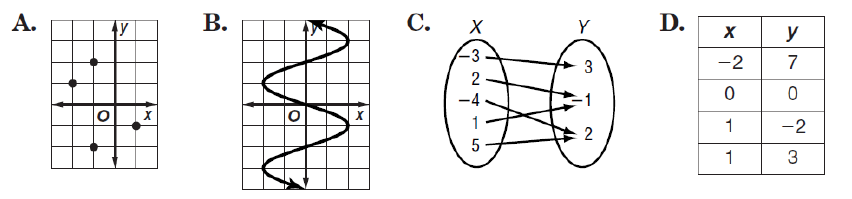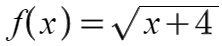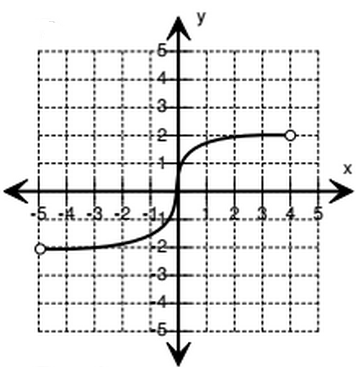### Day 03 - Parent Functions - 01.09.15

• Quiz 1 will be on Tuesday, January 13th
• Unit 1 Test will be on Tuesday, Jan. 20th
• Classbuilder
• Remind.com

Bell Ringer Quiz

1. What are functions?

1. a mathematical relationship in which any input has one and only one output

2. a mathematical relationship in which any input has two or more outputs

3. a mathematical relationship in which any input has at least one output

4. a mathematical relationship in which any output has at least one input

5. none of the above

2. Determine which relation is a function.3. If f(x) = x2 + 5x + 2, then find f(-2).

1. 4

2. -4

3. 2

4. -2

5. none of the above

4. What is the x and y-intercept of?

1. x-intercept: (4,0) | y-intercept: (0,2)

2. x-intercept: (0,4) | y-intercept: (2,0)

3. x-intercept: (-4,0) | y-intercept: (0,-2)

4. x-intercept: (16,0) | y-intercept: (0,2)

5. none of the above

5. Find the domain and range of the following:1. D: (-5,4) | R: (2,2)

2. D: (5,4) | R: (-2,2)

3. D: (-5,4) | R: (-2,2)

4. D: (5,-4) | R: (2,-2)

5. none of the above

Review
• Intro to Functions
• What is a function?
• Function Notation
• Evaluating Functions
• Examples/Counterexamples
• Graph
• Table
• Set
• Domain/Range (using functions students covered in Algebra: linear, constant, square root, etc.)
• Graph
• Table
• Set
• Using Words
• Compound Inequalities
• Interval Notation
• Intercepts

Lesson
• Intervals Of Increasing And Decreasing (Quadratic and Absolute Value Functions)
• Extrema
• Relative Min/Max (Cubic Functions)
• Absolute Min/Max (Quadratic and Absolute Value Functions)
• Using the graphing calculator to calculate mins and maxs
• Note: Address issue with rounding using the calculator
• Symmetry
• Axis of Symmetry (Quadratic and Absolute Value Functions)
• End Behavior
• Evaluating Functions
• Function Operations
• Composition

Exit Ticket
• Posted on board at the end of the block
Lesson Objective(s)
• How are parent functions related to other functions?
• How are function evaluated?

#### In-Class Help Requests

Standard(s)
• CC.9-12.F.IF.1 Understand the concept of a function and use function notation. Understand that a function from one set (called the domain) to another set (called the range) assigns to each element of the domain exactly one element of the range. If f is a function and x is an element of its domain, then f(x) denotes the output of f corresponding to the input x. The graph of f is the graph of the equation y = f(x).
• CC.9-12.F.IF.2 Understand the concept of a function and use function notation. Use function notation, evaluate functions for inputs in their domains, and interpret statements that use function notation in terms of a context.
• CC.9-12.F.IF.6 Interpret functions that arise in applications in terms of the context.  Calculate and interpret the average rate of change of a function (presented symbolically or as a table) over a specified interval. Estimate the rate of change from a graph.*
• CC.9-12.F.IF.7 Analyze functions using different representations. Graph functions expressed symbolically and show key features of the graph, by hand in simple cases and using technology for more complicated cases.*
• CC.9-12.F.IF.7a Graph linear and quadratic functions and show intercepts, maxima, and minima.*
• CC.9-12.F.IF.7b Graph square root, cube root, and piecewise-defined functions, including step functions and absolute value functions.*
• CC.9-12.F.IF.8 Analyze functions using different representations. Write a function defined by an expression in different but equivalent forms to reveal and explain different properties of the function.
• CC.9-12.F.IF.9 Analyze functions using different representations. Compare properties of two functions each represented in a different way (algebraically, graphically, numerically in tables, or by verbal descriptions). For example, given a graph of one quadratic function and an algebraic expression for another, say which has the larger maximum.
• CC.9-12.F.BF.1c (+) Compose functions.
• CC.9-12.F.BF.3 Build new functions from existing functions. Identify the effect on the graph of replacing f(x) by f(x) + k, k f(x), f(kx), and f(x + k) for specific values of k (both positive and negative); find the value of k given the graphs. Experiment with cases and illustrate an explanation of the effects on the graph using technology.
• CC.9-12.F.LE.2 Construct and compare linear, quadratic, and exponential models and solve problems. Construct linear and exponential functions, including arithmetic and geometric sequences, given a graph, a description of a relationship, or two input-output pairs (include reading these from a table).

Mathematical Practice(s)
• #1 - Make sense of problems and persevere in solving them
• #2 - Reason abstractly and quantitatively
• #4 - Model with mathematics
• #5 - Use appropriate tools strategically
• #7 - Look for and make use of structure

Past Checkpoints